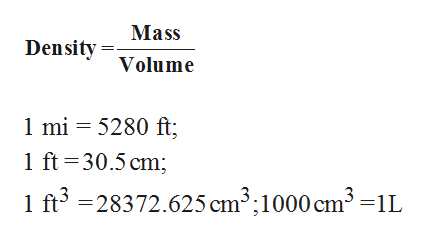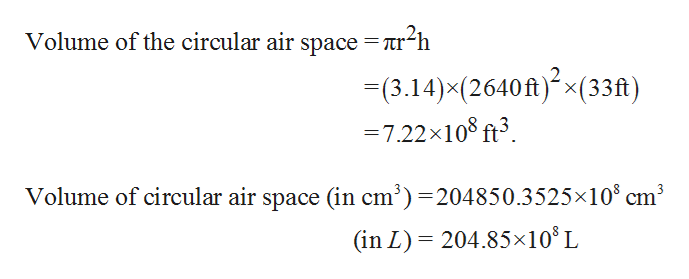# Be sure to answer all parts. Pheromones are compounds secreted by females of many insect species to attract mates. Typically, 1.00 × 10−8 g of a pheromone is sufficient to reach all targeted males within a radius of 0.500 mi. Calculate the density of the pheromone (in grams per liter) in a cylindrical air space having a radius of 0.500 mi and a height of 33.0 ft (volume of a cylinder of radius r and height h is πr2h). Enter your answer in scientific notation.

Question
 Be sure to answer all parts. Pheromones are compounds secreted by females of many insect species to attract mates. Typically, 1.00 × 10−8 g of a pheromone is sufficient to reach all targeted males within a radius of 0.500 mi. Calculate the density of the pheromone (in grams per liter) in a cylindrical air space having a radius of 0.500 mi and a height of 33.0 ft (volume of a cylinder of radius r and height h is πr2h). Enter your answer in scientific notation.
check_circleExpert Solution
Step 1

Density is the ratio of mass to Volume.

By using known unit conversion, the density is calculated.help_outlineImage TranscriptioncloseMass DensityVolume 1 mi 5280 ft; 1 ft 30.5 cm; 1 ft3-28372.625 cm3;1000 cm3 -1L fullscreen
Step 2

Volume of cylindrical air space is calculated as,help_outlineImage TranscriptioncloseVolume of the circular air space = ir-h 3.14)x(2640ft)(33ft) = 7.22x108 ft3 Volume of circular air space (in cm3)=204850.3525x108 cm3 204.85x108 L (in L) fullscreen

### Want to see the full answer?

See Solution

#### Want to see this answer and more?

Solutions are written by subject experts who are available 24/7. Questions are typically answered within 1 hour*

See Solution
*Response times may vary by subject and question
Tagged in

### Chemistry